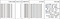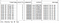“Without big data, you are blind and deaf and in the middle of a freeway.” — Geoffrey Moore

These days big data has become the most essential part of the computer world. One important part is to read and analyze a huge amount of logs generated by different types of databases or other services or products which we use. Since MongoDB has entered into our ecosystem, so it has now become vital to understand the mongo logs and extract some useful information from it. I read and researched about how to evaluate these crores of lines which led me to PySpark.

In this article, I am assuming that PySpark is already installed (if not installed, I have added URLs at the end of the article which will help you install the PySpark). MongoDB log messages are in the following format:

`<timestamp> <severity> <component> [<context>] <message>ex: 2019-07-08T06:26:01.021+0000 I CONTROL  [signalProcessingThread] Replica Set Member State: PRIMARY`

You can read more details about MongoDB log messages on this link. You will get to know more about messages and the regular expressions which we are going to build.
First of all load the MongoDB log file in the program

`sc = SparkContext()sqlContext = SQLContext(sc)spark = SparkSession(sc)base_df = spark.read.text("path_to_log_file")# base_df is now a spark data frameprint(base_df)                    # pyspark.sql.dataframe.DataFramebase_df1.count()                  # count number of logsbase_df1.show(10, truncate=True)  # showing first 10 logs`

We will be using regular expressions to segregate different sections in the message.

`timestamp_regex = "\d{4}-\d{2}-\d{2}T\d{2}:\d{2}:\d{2}.\d{3}[+]\d{4}"severity_levels_regex = "[F, E, W, I, D]\s"component_regex = "(ACCESS|COMMAND|CONTROL|FTDC|GEO|INDEX|NETWORK|QUERY|REPL|REPL_HB|ROLLBACK|SHARDING|STORAGE|RECOVERY|JOURNAL|TXN|WRITE)"context_regex = "\[(.*?)\]"message_regex = "\]([\s\S]*)"`

After this, we will be building up a tabular data frame on which can apply different filters and perform queries.

`logs_df = base_df1.select(regexp_extract('value', timestamp_regex, 0).alias('timestamp'),regexp_extract('value', severity_levels_regex, 0).alias('severity'),regexp_extract('value', component_regex, 0).alias('component'),regexp_extract('value', context_regex, 1).alias('context'),regexp_extract('value', message_regex, 1).alias('message'))print(logs_df)logs_df.show(10, truncate=True)`

Following is the output of the above two statements:
DataFrame[timestamp: string, severity: string, component: string, context: string, message: string]Output: logs_df.show(10, truncate=True)

Now, we have the complete file in a data frame so we can perform different operations like:

a. Since MongoDB logs each query which takes more than 100ms to execute. We can filter out the queries which are taking more time to execute. Following is the log of a query:

|2019–07–15T05:55:52.236+0000|I |COMMAND |conn24971548 | command <dbname>.<collection_name> command: find { find: “<collection_name>”, filter: { requestId: “0286aedf-e042–4961–8ffc-578847fabf15” }, projection: { _id: 0 }, limit: 1, singleBatch: true, lsid: { id: UUID(“70382cde-19a2e2f74fbfd”) }, \$clusterTime: { clusterTime: Timestamp(1563170121, 8), signature: { keyId: 665826205825, hash: BinData(0, 095B915FDE2D3597D708E54E) } }, \$db: <db_name>, \$readPreference: { mode: “primaryPreferred” } } planSummary: COLLSCAN keysExamined:0 docsExamined:175254 cursorExhausted:1 numYields:1397 nreturned:1 reslen:2271 locks:{ Global: { acquireCount: { r: 1398 } }, Database: { acquireCount: { r: 1398 } }, Collection: { acquireCount: { r: 1398 } } } storage:{ data: { bytesRead: 516242193, timeReadingMicros: 2598279 } } protocol:op_msg 3666ms

As you can see in the log, time is logged at the end of the statement, we can use this time to filter out such queries. We can use following function to filter out the queries which are taking longer than 3000ms (change according to your requirement). Also, the ‘component’ in these types of logs is equal to “COMMAND” or “WRITE”, therefore, we need to filter out these two components also.

`# Function to filter queries taking longer than 3000msdef eval_string(string):    time_taken = string.split(' ')[-1]    if time_taken[:-2].isdigit() and int(time_taken[:-2]) > 3000:        return string    return ""# Filtering out the COMMAND and WRITE components from data framecomponents = logs_df[ ((logs_df['component'] == "COMMAND") | (logs_df['component'] == "WRITE")) ]# udf is used to create a user defined functionudf_myFunction = udf(eval_string) # if the function returns an intdf = components.withColumn("message", udf_myFunction("message"))# eval_string will return "" in case of time<3000ms, # filter out the queries in which message is not equal to ""df.filter(df.message != "").show(10, truncate=True)`Showing 10 rows of filtered queries. Mention truncate=False to see complete queries

Following is the complete program:

`from pyspark.context import SparkContextfrom pyspark.sql.context import SQLContextfrom pyspark.sql.session import SparkSessionfrom pyspark.sql.functions import regexp_extract, lit, col, udffrom pyspark.sql import DataFrameimport refrom functools import reduceimport jsondef eval_string(string):    time_taken = string.split(' ')[-1]    if time_taken[:-2].isdigit() and int(time_taken[:-2]) > 3000:        return string    return ""sc = SparkContext()sqlContext = SQLContext(sc)spark = SparkSession(sc)base_df = spark.read.text("/home/himanshu/Downloads/mongolog/mongod.log.1")timestamp_regex = "\d{4}-\d{2}-\d{2}T\d{2}:\d{2}:\d{2}.\d{3}[+]\d{4}"severity_levels_regex = "[F, E, W, I, D]\s"component_regex = "(ACCESS|COMMAND|CONTROL|FTDC|GEO|INDEX|NETWORK|QUERY|REPL|REPL_HB|ROLLBACK|SHARDING|STORAGE|RECOVERY|JOURNAL|TXN|WRITE)"context_regex = "\[(.*?)\]"message_regex = "\]([\s\S]*)"logs_df = base_df1.select(regexp_extract('value', timestamp_regex, 0).alias('timestamp'),regexp_extract('value', severity_levels_regex, 0).alias('severity'),regexp_extract('value', component_regex, 0).alias('component'),regexp_extract('value', context_regex, 1).alias('context'),regexp_extract('value', message_regex, 1).alias('message'))# Show in desc order according to timestamp logs_df.sort(col("timestamp").desc()).show(10, truncate=True)logs_df.show(10, truncate=True)components = logs_df[ ((logs_df['component'] == "COMMAND") | (logs_df['component'] == "WRITE")) ]udf_myFunction = udf(eval_string) # if the function returns an intdf = components.withColumn("message", udf_myFunction("message"))df.filter(df.message != "").show(10, truncate=True)# Count the filtered logsdf.filter(df.message != "").count()`

Since we have the complete data frame, we can filter out logs according to our requirements. Do let me know if there are any queries for filtering out the logs or any other issues. Also, comment if I can improve this or if there is an issue in the program.

## HackerNoon.com

#### how hackers start their afternoons.

Welcome to a place where words matter. On Medium, smart voices and original ideas take center stage - with no ads in sight. Watch
Follow all the topics you care about, and we’ll deliver the best stories for you to your homepage and inbox. Explore
Get unlimited access to the best stories on Medium — and support writers while you’re at it. Just \$5/month. Upgrade